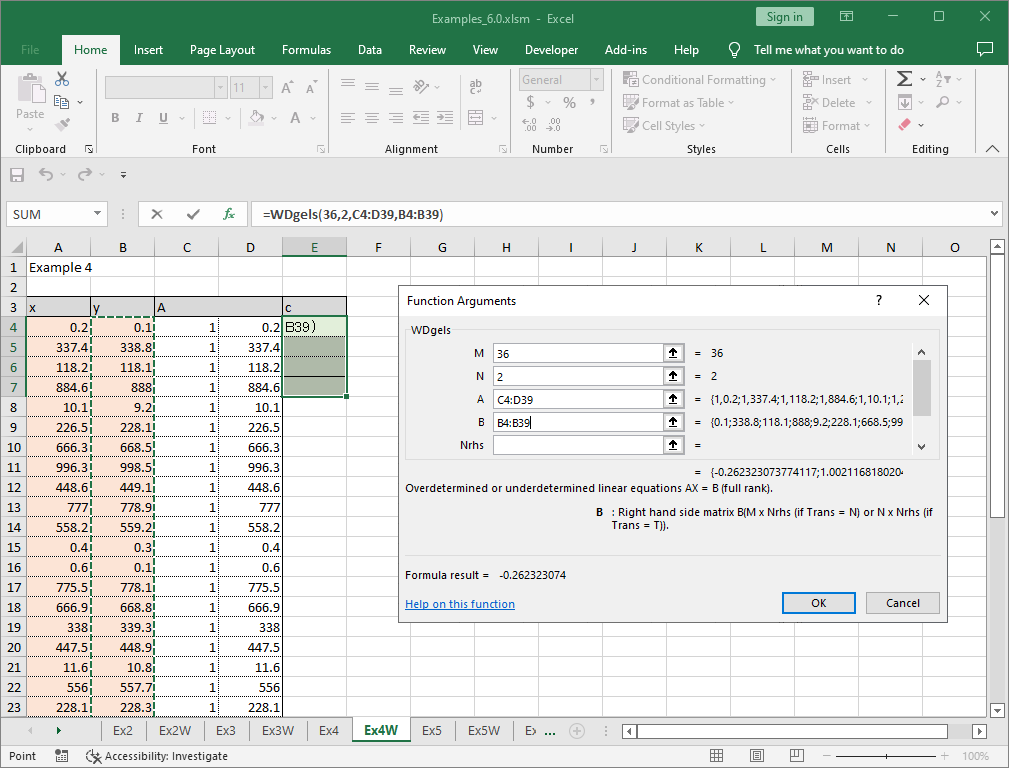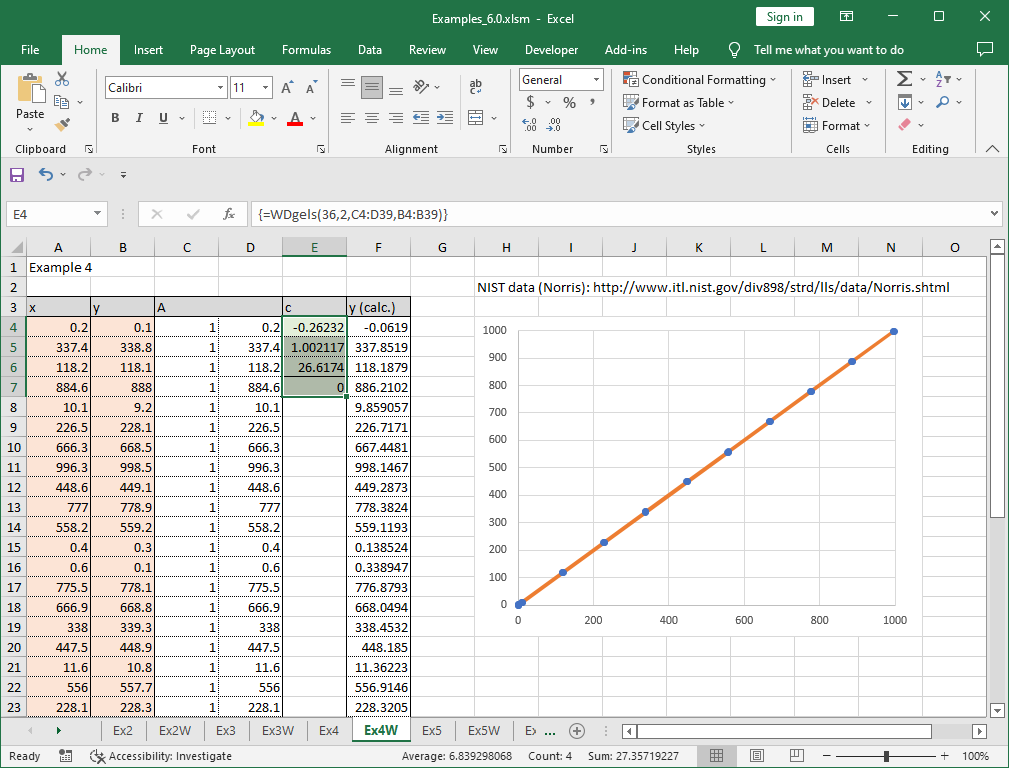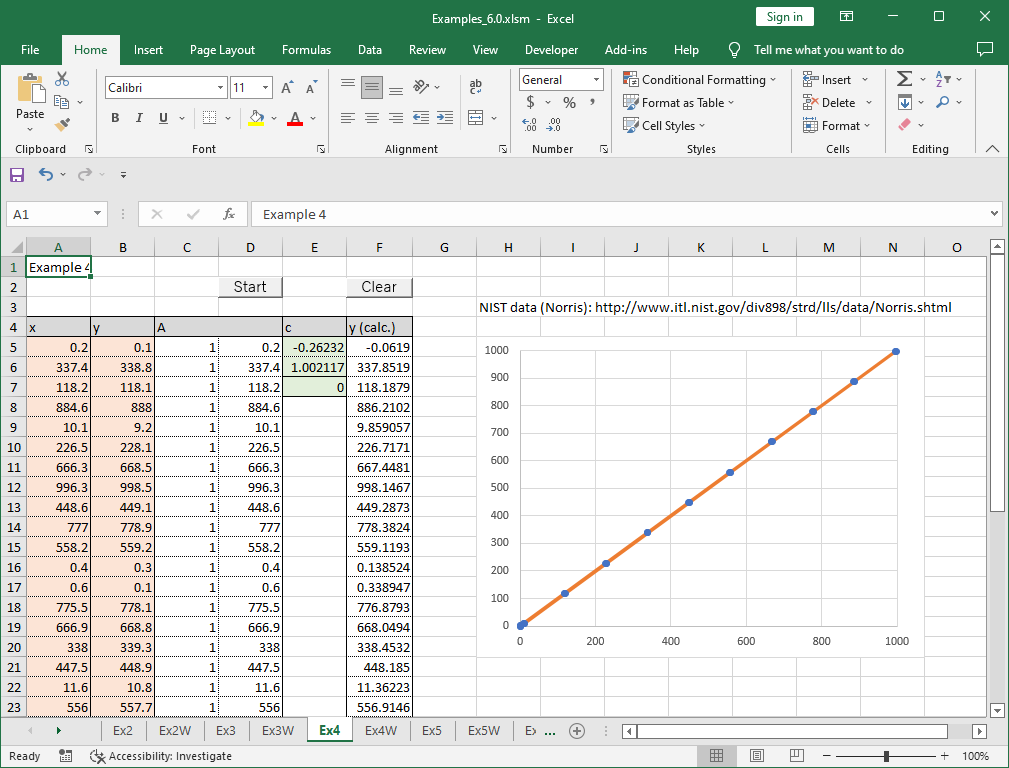# 4. Linear least squares problems

Note – The example worksheets are available for download. See 1. How to obtain and use example worksheets.

Examples of solving linear least squares problems by XLPack are shown below.

### Example: Polynomial approximation

The following data is the test data of NIST (National Institute of Standards and Technology) which can be found at the web site http://www.itl.nist.gov/div898/strd/lls/data/Norris.shtml

```
Data:     y          x
0.1        0.2
338.8      337.4
118.1      118.2
888.0      884.6
9.2       10.1
228.1      226.5
668.5      666.3
998.5      996.3
449.1      448.6
778.9      777.0
559.2      558.2
0.3        0.4
0.1        0.6
778.1      775.5
668.8      666.9
339.3      338.0
448.9      447.5
10.8       11.6
557.7      556.0
228.3      228.1
998.0      995.8
888.8      887.6
119.6      120.2
0.3        0.3
0.6        0.3
557.6      556.8
339.3      339.1
888.0      887.2
998.5      999.0
778.9      779.0
10.2       11.1
117.6      118.3
228.9      229.2
668.4      669.1
449.2      448.9
0.2        0.5
```

Let’s try to fit a polynomial of degree 1 (straight line approximation).

```
f(x) ≅ c1 + c2x

```

Two solving methods using worksheet function WDgels and VBA subroutine Dgels are shown below.

### Solving problem with worksheet function

Enter the data X and Y into the appropriate cells (orange cells) in the worksheet and compute matrix A (Aij = φj(xi) (i = 1 to m, j = 1 to n)) (in this case, enter formulas that give Ai1 = 1 and Ai2 = xi). Then select (n + 2) cells where the solution and other results are to be entered (green cells) and enter woeksheet function WDgels.

The parameters required by WDgels are Trans, Cov, M, N, A, B and Nrhs. Trans is “N” (solve Ax = b) or “T” (solve (A^)x = b). Cov is “N” (do not compute variance-covariance matrix), “D” (compute variance only) or “C” (compute variance-covariance matrix)). M is the number of data points, N is the number of parameters, A is the cell range of the matrix A, and B is the cell range of the right-hand side vector (= vector y). The right-hand side vector is one in most cases. However, more than one vectors can be entered and Nrhs is the number of right-hand side vectors. Nrhs can be omitted, in which case it is assumed to be one.Then press Ctrl + Shift + Enter. The parameters are obtained in the selected cells.

In this example, the graph is also created using the calculation result. The input data are shown by the circles and the calculation result is shown by the line.Now, we’ve got the parameters c1 = -0.26232 and c2 = 1.002117. These values are exactly same with the values of NIST.

### Solving problem with VBA program

Let’s solve the same example problem by VBA program. The following is an example code using VBA library subroutine Dgels.

``````Sub Start()
Const MMax = 100, NMax = 5
Dim M As Long, N As Long
Dim A(MMax, NMax) As Double, B(MMax) As Double
Dim Info As Long, I As Long, J As Long
'--- Input data
M = 36: N = 2
For I = 0 To M - 1
For J = 0 To N - 1
A(I, J) = Cells(5 + I, 3 + J)
Next
B(I) = Cells(5 + I, 2)
Next
'--- Compute least squares solution
Call Dgels("N", M, N, A(), B(), Info)
'--- Output result
For J = 0 To N - 1
Cells(5 + J, 5) = B(J)
Next
Cells(7, 5) = Info
End Sub``````

After entering the data into the cells, the same result was obtained by running the macro “Start” as follows. Unlike the example using worksheet functions, you cannot obtain results by simply entering the values of matrix A and vector y, and you need to run a VBA program.